Maths-
General
Easy

Question

# If the sides of a triangle are in the ratio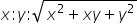then greatest angle is

##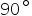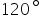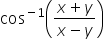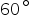Hint:

## The correct answer is:### As the ratio of the sides isa= x, b=y, c =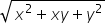c is the largest side, so, C is the largest angle.So, the angle of the greatest angle is 120.#### With Turito Foundation.#### Get an Expert Advice From Turito.